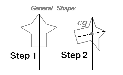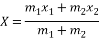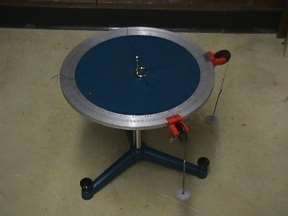Disclaimer: This is an example of a student written essay.

Any scientific information contained within this essay should not be treated as fact, this content is to be used for educational purposes only and may contain factual inaccuracies or be out of date.

# Conditions for Equilibrium Experiment

 ✅ Paper Type: Free Essay ✅ Subject: Physics ✅ Wordcount: 1527 words ✅ Published: 12th Mar 2018

Laboratory Report

Tricia Desierto, Luis Diaz, Karhen Estella, Gabrielle Beatrix Francisco

Department of Biological Science

College of Science, University of Santo TomasEspanÌƒa, Manila, Philippines

Abstract

The object is said to be in a state of equilibrium, when the forces acting upon an object are balanced. There were four activities done in the experiment. In the first activity the equilibrant force was determined. The second activity, unknown forces was determined. For the third activity,center of gravity was located. The last activity, rotational equilibrium was demonstrated.

I. Introduction

Equilibrium is moving with constant velocity. It is a condition that the rotational motion of the body may also remain constant. A body is in equilibrium or at rest only when there is no movement or rotation done. When the resultant force acting on the object is zero the object is in equilibrium. The objectives of the experiment are: to determine the equilibrant force by using the component and table method;to determine the unknown forces using the first and second conditions for equilibrium; to locate the center of gravity of a composite body;and to demonstrate the rotational equilibrium.

II. Theory

A situation wherein the net force acting on a certain object is zero and an object that has no motion or undergoes no rotational and traditional accelerationis said to be in a state of equilibrium wherein net torque and net force on the object is zero in all directions. For an object to be in equilibrium, two conditions should be met.

If you need assistance with writing your essay, our professional essay writing service is here to help!

The first condition tells us that the net force acting on the object needs to be zero which only means that for a certain axis of motion, the forces acting along that particular axis should sum up to zero.The second condition needed to attain equilibrium, on the other hand, involves avoiding or neglecting accelerated rotation and it should maintain a constant angular velocity. A rotating body can attain equilibrium if the rate of its rotation remains unchanged by the forces acting on that certain object.

The center of gravity is a geometric property of any object. It is the average location of the weight of an object. The motion of any object can be described through space in terms of the translation of the center of gravity of the object from one place to another and the rotation of the object about its center of gravity when it is free to rotate.Figure 1. Determination of the Center of Gravity using plumb line techniqueX= Center of Gravity m=Mass x= distance from a fixed point

Equation 1.Center of Gravity Formula

When an object is said to be in equilibrium, it is not moving or rotating. The pivotal axis can be any point outside or inside the object. The object’s linear and angular accelerations are both zero and the sum of the torquesacting on a system should be equal to zero.The sum of the counter-clockwise torques should be equal to the sum of the clockwise torques.

III. MethodologyActivity 1: Equilibrant Force

Three pans labelled as A, B and C was weighed. Pans A and B were hanged respectively at the 300 and 2000 marks on the force table. 100g was placed on pan A and 150g on pan B. The tension acting on the string, the weight of the pan plus the weight added to the pan was recorded as TA andTB respectively. The two tensions in the strings were balanced by placing weight on pan C or adjusting its position. The tensions are balanced if the pin is exactly at the center of the ring. The magnitude of the equilibrant, the weight of pan C plus the weight added to it, and its position was recorded. The theoretical equilibrant of the two tensions was determined by component method and the % error was computed.

Activity 2: First Condition for Equilibrium

A cylinder of unknown weight was suspended using the force board by means of two strings. A spring scale was attached to one of the strings and was pulled horizontally until the pin on the force board was exactly at the middle of the ring. The reading on the spring scale was recorded as T1. The angle that the other string made was recorded as θ. A free body diagram of the ring was drawn. The tension of T2 in the other string and the weight of the cylinder were solved. The cylinder was weighed for the accepted value and the % error was computed.

Activity 3: Locating the Center of Gravity

A circle with a diameter of 10cm and a square with a side of 10cm were cut out from a card board. The weights of WC and WS were determined. The center of gravity of the composite figure was determined by balancing method and plumb line method. The position of the center of gravity was specified using the leftmost side of the square as the y-axis and the bottom square as the x-axis. The results were checked by actual computation for the center of gravity.

Activity 4: Second Condition for Equilibrium

The center of gravity of an aluminium bar was located by balancing it on a pencil and the position for the center of gravity was marked. The cylinder used in the previous activity was hanged 5.0cm from one end of the bar. Using the force board, the aluminium bar was supported by means of a spring scale on one end and a string on the other end until the bar assumed a horizontal position. A free body diagram of the bar was drawn. The second condition for equilibrium was used to determine the weight of the bar and the tension in the string. The theoretical weight of the cylinder was used in the computation. The bar was weighed for the accepted value and the % error was computed

IV. Results and Discussion

V. Conclusion

The equilibriant force was successfully determined using the component and table method, with an acceptable value for the % error – 8.70% and 4.47%.

The unknown forces were also determined using the first condition of equilibrium with a % error of only 4.57%

The center of gravity was defined more accurately with the Plumb Line Method as opposed to the Balancing Method.

The unknown forces were unsuccessfully defined using the second condition of equilibrium, as the % error exceeds the acceptable range at 51.76%.

VI. Applications

VII. References

Lesson24:Equilibrium. (n.d.). Retrieved December 8, 2013, from studyphysics: http://www.studyphysics.ca/newnotes/20/unit01_kinematicsdynamics/chp06_vectors/lesson24.htm

First Condition. (n.d.). Retrieved December 8, 2013, from boundless: https://www.boundless.com/physics/static-equilibrium-elasticity-and-torque/conditions-for-equilibrium/first-condition/

Second Condition. (n.d.). Retrieved December 8, 2013, from Boundless: https://www.boundless.com/physics/static-equilibrium-elasticity-and-torque/conditions-for-equilibrium/second-condition/

Rotational Equilibrium. (n.d.). Retrieved December 8, 2013, from faculty: http://faculty.wwu.edu/vawter/PhysicsNet/Topics/RotationalDynamics/RotEquilibrium.html

Benson, T. (2008, July 18). Center of gravity. Retrieved December 8, 2013, from grc: http://www.grc.nasa.gov/WWW/k-12/airplane/cg.html

View all

## DMCA / Removal Request

If you are the original writer of this essay and no longer wish to have your work published on UKEssays.com then please:

Related Services

Prices from

BHD57

Approximate costs for:

• 1000 words
• 7 day deliveryHumanity University

Dedicated to your worth and value as a human being!

Related Lectures